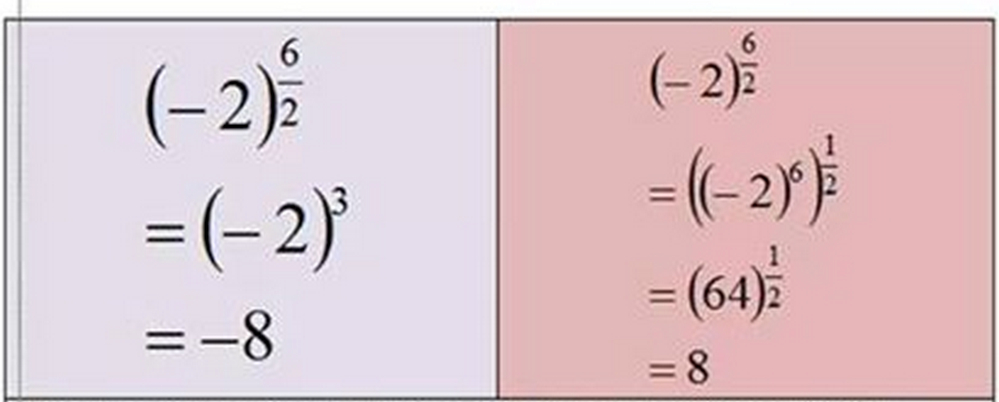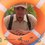# Why it is different?Anybody can explain why the results are different?Note by Mas Mus
6 years, 8 months ago

This discussion board is a place to discuss our Daily Challenges and the math and science related to those challenges. Explanations are more than just a solution — they should explain the steps and thinking strategies that you used to obtain the solution. Comments should further the discussion of math and science.

When posting on Brilliant:

• Use the emojis to react to an explanation, whether you're congratulating a job well done , or just really confused .
• Ask specific questions about the challenge or the steps in somebody's explanation. Well-posed questions can add a lot to the discussion, but posting "I don't understand!" doesn't help anyone.
• Try to contribute something new to the discussion, whether it is an extension, generalization or other idea related to the challenge.
• Stay on topic — we're all here to learn more about math and science, not to hear about your favorite get-rich-quick scheme or current world events.

MarkdownAppears as
*italics* or _italics_ italics
**bold** or __bold__ bold
- bulleted- list
• bulleted
• list
1. numbered2. list
1. numbered
2. list
Note: you must add a full line of space before and after lists for them to show up correctly
paragraph 1paragraph 2

paragraph 1

paragraph 2

[example link](https://brilliant.org)example link
> This is a quote
This is a quote
    # I indented these lines
# 4 spaces, and now they show
# up as a code block.

print "hello world"
# I indented these lines
# 4 spaces, and now they show
# up as a code block.

print "hello world"
MathAppears as
Remember to wrap math in $$ ... $$ or $ ... $ to ensure proper formatting.
2 \times 3 $2 \times 3$
2^{34} $2^{34}$
a_{i-1} $a_{i-1}$
\frac{2}{3} $\frac{2}{3}$
\sqrt{2} $\sqrt{2}$
\sum_{i=1}^3 $\sum_{i=1}^3$
\sin \theta $\sin \theta$
\boxed{123} $\boxed{123}$

## Comments

Sort by:

Top Newest

The results of raising things into power strictly depends on the writer's interpretation; whereas $\frac{6}{2}=3$, raising to $\frac{6}{2}$ is not the same as raising to $3$. First, let's focus on $\frac{6}{2}$.

This is because there's a difference between $\sqrt{(-2)^6}$ and $(\sqrt{-2})^6$.

(i) In one, you're ordering the operation raise this value into the sixth power and square root it

(ii) In another, you're ordering the operation square root this value and raise it into the sixth power.

Here's how they differ: In (i), you will be taking a square root of a single value, and will thus obtain two distinct roots (unless it's 0). In (ii), you will be finding the two distinct square roots, and then raising each of them individually into the sixth power. If your answer was in a complex form, or were being raised into an odd power, you would actually get two distinct answers upon raising into that power; but either way, the results shall differ.

Now, raising to $3$ is another story. When you choose to simplify $\frac{6}{2}$ to $3$, you're killing off roots. Take a look at the following example:

$x^2=x$.

Now, you may be tempted to cancel the $x$ on both sides and cheer $x=1$!, but then you'd be killing a root:

$x^2-x=0$

$x(x-1)=0$

$\boxed{x=0,1}$

(iii) Here, you're ordering the operation raise this value into the third power

In conclusion, it's just a matter of notation. This is one of those confusing wings of mathematics that teachers and professors leave out in high schools and colleges. And this is why generally mathematicians refer to "principal roots." But all of this jibber-jabr isn't for nothing. In real-world applications, depending on what you're trying to calculate, you may want to take the square root of a value to the sixth power to observe symmetry, or just cube it because your model is simplistic. It's all up to you.

Cheers,

- 6 years, 8 months ago

Log in to reply

The right one is :>

$(-2)^{\frac{6}{2}}$

$=(-2)^{3}$

$=-8$

But the question is why the other one is wrong.

I will try to explain it ::

$(-2)^{\frac{6}{2}}$

$=((-2)^{6})^{\frac{1}{2}}$ No doubt !!! This step is absolutely right.

$=\sqrt{(-2)^{6}}$

$=\sqrt{ (-2).(-2).(-2).(-2).(-2).(-2)}$

$=\sqrt{-2}.\sqrt{-2}.\sqrt{-2}.\sqrt{-2}.\sqrt{-2}.\sqrt{-2}.$

$=\sqrt{2}.i \sqrt{2}.i \sqrt{2}.i \sqrt{2}.i \sqrt{2}.i \sqrt{2}.i$ (Here $i=\sqrt{-1}$ ,i.e. iota )

$=(\sqrt{2})^{6} . i^{6}$

$=8 \times -1$ (Because * $i^{6}=i^{4}.i^{2}=1 \times -1=-1)$ *

Hidden concept here :

$\sqrt{a} \times \sqrt{b}=\sqrt{ab}$ if and only if, at least one of a and b is positive

If both a and b are negative, then:

$\sqrt{a} \times \sqrt{b}=\sqrt{|a|}.i \times \sqrt{|b|}.i = i^{2}. \sqrt{|a|} \times \sqrt{|b|}=-\sqrt{|a|.|b|}$

- 6 years, 8 months ago

Log in to reply

Hm that's interesting! But I'm afraid the operation you're applying when splitting the squareroots is $(\sqrt{-2})^6$, so you're changing the operation in a way. In $\sqrt{(-2)^6}$, you're asked to root $(-2)^6$, i.e. the finished product, or the positive value.

But anyway, what you're doing is absolutely consistent if you consider only the principal root. In this case, it is $-8$. But if you're to consider all the roots, you'd have to factor in the negative counterpart, which is killed the second you define $((-2)^6)^{\frac{1}{2}}=\sqrt{(-2)^6}$.

So yeah. By FTA, $\pm 8$. And this is more apparent if you consider $x^{2/4}$. This should be the same as $x^{1/2}$, right? But if you observe carefully, you'll notice that with $x^{2/4}$ you'll ultimately end up with either four distinct roots (if you choose to root last) or one root, whereas in $x^{1/2}$ you're guaranteed two ($x\neq 0$).

But that "hidden concept" at the end... requires more thought from my end. Thanks for sharing.

Cheers,

- 6 years, 8 months ago

Log in to reply

Root of a square(or even power) always comes out with modulus

$\sqrt{x^{2}} = |x|$

- 6 years, 8 months ago

Log in to reply

Why's that? If $x=-2,$ $x^2=4$. But according to your expression, $\sqrt{x^2}=2$. But we know $x=-2$.

Thus, $\sqrt{x^2}=\pm x$.

Let's have another look:

$(-2)^2=4$.

$\sqrt{(-2)^2}=\sqrt{4}=\pm 2$.

Since $x\neq +2$, $\boxed{x=-2}$.

($\pm \rightarrow$ plus OR minus).

Cheers,

- 6 years, 8 months ago

Log in to reply

$\sqrt{(-2)^2}$ is not equal to $-2$.

$\sqrt{(-2)^2}$ is equal to $2$.

This symbol that you're using, $\sqrt{\quad}$, is called the principle square root operator. What it does is it takes a a non-negative integer and spits out its principal square root.

$\sqrt{x^2}$ is not always equal to $x$.

- 6 years, 8 months ago

Log in to reply

Oh really? I never knew that! I knew about principal square roots, but thought they were only subjective to the problem.

Well what a discovery!

But still $x^{\frac{1}{2}}$ is not taking the principal square root, but it is taking both roots. So I just used the square root symbol as a way to show it.

Thanks for pointing that out, though.

- 6 years, 8 months ago

Log in to reply

The function $f(x)=\sqrt{x}$ has its domain in the non-negative reals. $\sqrt{x^2}=|x|$, so @Krishna Sharma is right.

- 6 years, 8 months ago

Log in to reply

Yes, it does. But taking square roots of specific numbers is not a function, it's an operation. A function is one system of expressing the results of operations. So in its full color, we'd actually be looking at $y^2=x$, which is a relation, and thus we'd have a tilted parabola, with both positive and negative outputs.

Besides, you should know this from the Fundamental Theorem of Algebra: a polynomial with degree $n$ has $n$ roots. Since the polynomial we're interested in is $y^2$ (whose "roots" we're taking), it will have two roots.

If you'd like a more complete explanation, let me know.

Cheers,

- 6 years, 8 months ago

Log in to reply

sir , i wish to hear a complete explanation ....... still confused ; hows that the solution set achieved in first case, got confined to { -8 } and in second case it is {+8 , -8 } ?

- 6 years, 7 months ago

Log in to reply

Hello,

If you read what I've written above (the top comment), you can see that both of the transformations are right, but we're just "ordering" different operations. The comment right below mine provides a strong counterargument against this, and it may be right, but the fact that in applied physics we may sometimes want to specifically take $n$ amount of roots of a quantity raised to some sort of power is unchanged.

- 6 years, 7 months ago

Log in to reply

Thanks

- 6 years, 7 months ago

Log in to reply

These results are differents because ${ \left( -2 \right) }^{ \frac { 6 }{ 2 } }\neq { \left( { \left( -2 \right) }^{ 6 } \right) }^{ \frac { 1 }{ 2 } }$.

${ \left( { \left( -2 \right) }^{ 6 } \right) }^{ \frac { 1 }{ 2 } }=\sqrt { { \left( -2 \right) }^{ 6 } }$ ) not ${ \sqrt { \left( -2 \right) } }^{ 6 }$.

The correct way to solve this in the 2nd step in the pink box is ${ \left( { \left( -2 \right) }^{ \frac { 1 }{ 2 } } \right) }^{ 6 }$because ${ \left( { \left( -2 \right) }^{ \frac { 1 }{ 2 } } \right) }^{ 6 }={ \sqrt { \left( -2 \right) } }^{ 6 }={ \left( -2 \right) }^{ \frac { 6 }{ 2 } }$

- 6 years, 8 months ago

Log in to reply

when you square by odd the negative sign still stays and is possible. However, once you power by an even number, and the number will always be positive no matter what other number power you put it to after. Hence, the parenthesis should be around 6/2 because that should be done first, so the -2 isnt powered by an even exponent.

- 6 years, 8 months ago

Log in to reply

Another thing, when you take square root you should have two possible answers....8 and -8. b/c both squared gives you 64. So it works but the 8 is extraneous and you missed the -8

- 6 years, 8 months ago

Log in to reply

i dun get it , the pink one box :O

- 6 years, 8 months ago

Log in to reply

simply "BODMAS" first division then multiplication . In second case also we have divide first i.e., (1/2) then multiply...........

- 6 years, 8 months ago

Log in to reply

in (-2)^6/2 the square root after multiplying to power 6 may be +ve or -ve

- 6 years, 8 months ago

Log in to reply

You might've not concentrated enough. Look the right side of your question there you've written - 64 to the power 1/2 This is the same as square root of 64.

So at last you got your obvious answer as +/- 8. This is according to algebraic rules. Although you've not mentioned -8 there and written only +8.

- 6 years, 8 months ago

Log in to reply

(√-2)^6 =√2i^6 and while doing this directly we are missing iota I so there will be a difference

- 6 years, 8 months ago

Log in to reply

×

Problem Loading...

Note Loading...

Set Loading...